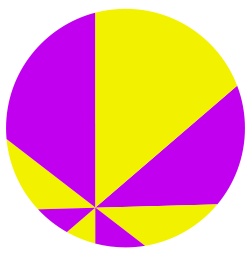# Global AI and Data Science

View Only

## THE PROBLEM :  In elementary geometry, the pizza theorem states the equality of two areas that arise when one partitions a disk in a certain way.

Let p be an interior point of the disk, and let n be a multiple of 4 and greater than or equal to 8. Form n sectors of the disk with equal angles by choosing an arbitrary line through p, rotating the line n/2 − 1 times by an angle of 2π/n radians, and slicing the disk on each of the resulting n/2 lines. Number the sectors consecutively in a clockwise or anti-clockwise fashion. Then the pizza theorem states that:

The sum of the areas of the odd-numbered sectors equals the sum of the areas of the even-numbered sectors .The pizza theorem is so called because it mimics a traditional pizza slicing technique. It shows that, if two people share a pizza sliced in this way by taking alternating slices, then they each get an equal amount of pizza.

## THE PROOFHISTORY :  The pizza theorem was originally proposed as a challenge problem by Upton (1967). The published solution to this problem, by Michael Goldberg, involved direct manipulation of the algebraic expressions for the areas of the sectors. Carter & Wagon (1994) provide an alternative proof by dissection. They show how to partition the sectors into smaller pieces so that each piece in an odd-numbered sector has a congruent piece in an even-numbered sector, and vice versa. Frederickson (2012) gave a family of dissection proofs for all cases (in which the number of sectors is 8, 12, 16, ...).

QUESTION I : Could there be a proof of this theorem using calculus?

QUESTION II : Could this theorem be generalized to sphere?

REFERENCE : Pizza Theorem – Wikipedia

#GlobalAIandDataScience
#GlobalDataScience